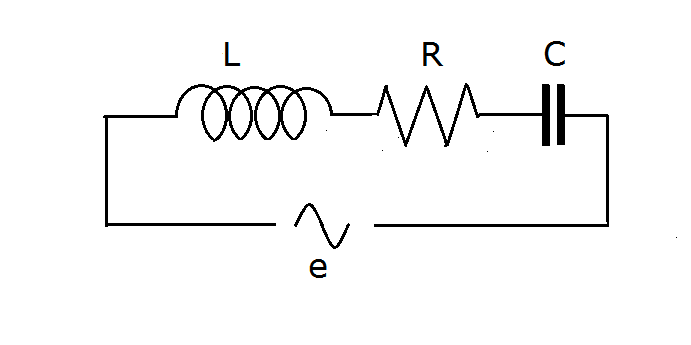# What Makes This Circuit Go?In a single loop, ac circuit voltage and current is given by:

$V(t) = 300 \sin ( 100 \pi t + \frac{\pi}{6})$
$I (t) = 5 \sin (100 \pi t - \frac{\pi}{6} )$

The circuit may be comprised of:

Note: The above image does not necessarily represent the circuit in the question.

×